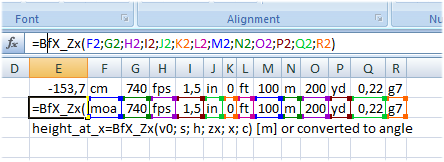# F o r u m

Home Ballistics for Excel Visit the BfX f o r u m
Home
Install/Update
Excel/VBA settings
Entering formulas
Drag functions
Robert Meijer

# Locale

The screenshots on this website have been made on computers having a Dutch locale. 2,3 in Dutch (and many other European countries) is the same as 2.3 elsewhere (UK, US, AS, ...). This impacts on how formulas in Excel cells are displayed. In my examples arguments in cell formulas are separated with semicolons ";" and I separate decimals with a comma ",". On many other computers one should use a comma "," as a value separator in Excel formulas and a decimal point "." in numbers.

• =bfx_d(740;40;0,5) (Netherlands and other European countries)
• =bfx_d(740,30,0.5) (UK, US, AU, ...)
Please be aware that BfX does not switch to Imperial or metric (SI) units automatically. This has to be done explicitly in every cell, see below. Some of the Excel spreadsheets I provide contains Visual Basic for Applications (VBA) code (computer programs). In VBA always the "." is used as decimal separator.

# The International System of Units (aka Metric aka SI units) and Imperal Units

If you not specify otherwise for every number you put in a formula in cell, BfX treats the number as being a value within the International System of Units. There is one exception, the ballistic coefficient is always in imperial units [lbs/inch2]. BfX supports however many imperial units also, read on.

# Entering formulas in Excell cells

The following picture illustrates how BfX works in Excel.BfX_Zx calculates the path heigth at a certain down range distance. In cell E3 the formula `=BfX_Zx()` is entered, no additional arguments. BfX returns then help on that function, here

```height_at_x=BfX_Zx(v0; s; h; zx; x; c) [m] or converted to angle```

where
• v0 [m/s] is the muzzle velocity
• s [m] is the height of the line of sight above the muzzle
• h [m] (usually 0) is the height of impact at the distance zx
• zx [m] is the distance where the impact is known (e.g. the scope zero distance)
• x [m] is the distance at which we want to know the path height
• c is the G1 ballistic coefficient in [lb/inch2] to be used with the Pejsa drag function
• height_at_x is then calculated in [m]
If not specified otherwise units belonging to the International System of Units (aka metric aka  SI). However, one can change the units of all variables. Row 1 and 2 shows the use of BfX_Zx with all parameters specified in the cells F1:R1 and F2:R2. To change the unit of the output variable, specify the unit in the first argument. In the picture above, this unit is "cm" in cell F1 and "moa" in cell F2. In the latter case, BfX converts the path height (in the example -153,7 [cm]) to minutes of angle. To specify an input unit, place that units behind the value, e.g. 200 [yd] in the example above. Ballistic coefficients in [kg/m2] are not accepted. Hence the ballistic coeficient c can only be followed by its drag function.

BfX supplies extensive help on its use in through  `=BfX_Help(n)` with n=0, 1, .... 130, click here for a listing. Similarly, `=BfX_Help2(n)` with n=0, 1, .... 100, lists all supported units and drag functions, look here.

It is not neccessary to specify the default units of an argument, one can omit them in the argument list of BfX functions.